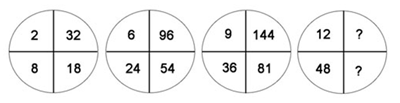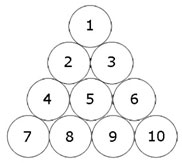# Missing Letters

Find the missing letters which complete the group.

B, C, D, E, ?, P, T, ?

# Complete The Series

B C D E __ I K __ X

The puzzle might look like a simple one. Give it a try.

# Missing Numbers

What are the missing numbers?# Number Puzzle

Make 100 using six 9. You can use addition, division and fraction.

# July Equals To…

If March = 43 and May = 39, what does July equals to?

# Quick and Easy Puzzle

Find the values of  a and b:

12 x a + b = 98

123 x a + b+1 = 987

1234 x a + b+2 = 9876

12345 x a + b+3 = 98765

123456 x a + b+4 = 987654

1234567 x a + b+5 = 9876543

12345678 x a + b+6 = 98765432

123456789 x a + b+7 = 987654321

# What Is The Next Number?

Fill in the sequence:

1   11   21   1211   111221  __________

Looks simple. Try solving it.

Do not post answers. Just write if you could answer it or not and how long did it take to solve.

# Invert The Triangle With Three Moves

By changing the position of only three circles, rearrange the triangle upside down so that the base is on top and the point is at the bottom.